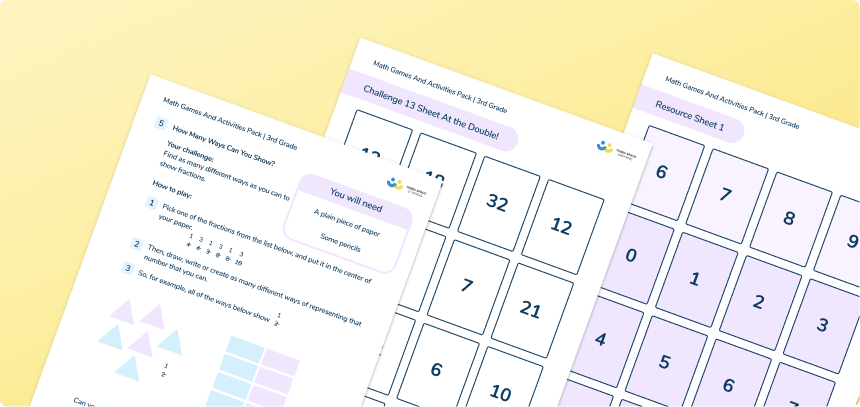Math teaching support you can trustone-on-one tutoring sessionsschools supported

[FREE] Fun Math Games and Activities Packs for Kindergarten to Grade 5

Ready to go, printable packs that teachers can use in the classroom.

# What Is A Unit Fraction? Explained For Teachers, Parents And Kids

In this post we will be explaining what a unit fraction is, what they mean, and providing you with some questions you can use to test a child’s skills.Fun Math Games and Activities Packs for Grade 5 - KindergartenDownload Free Now!

### What is a unit fraction?

A unit fraction is any fraction with 1 as its numerator (top number), and a whole number for the denominator (bottom number).

### When will children learn about them?

Although the terminology of ‘unit fraction’ is not yet introduced, 1st grade students should:

• Split circles and rectangles into two equal shares, describe the shares using the word ‘halves’ and use the phrase, “half of…”.
• Split circles and rectangles into four equal shares, describe the shares using the words “fourths” and “quarters, and use the phrases, “fourth of…” and “quarter of…”.
• When decomposing into more equal shares, it creates smaller shares (equal pieces)

In Second Grade, students use fractions to continue recognizing that equal shares of identical wholes don’t always have to have the same shape.

They are also introduced to thirds and are able to describe a whole as two halves, three thirds and four fourths.

• Develop understanding of fractions as numbers; understand a fraction a/b as the quantity formed by a parts of size 1/b.
• Explain equivalence of fractions, and compare fractions by reasoning about their size, using number lines and fraction models;
• compare and order fractions, and fractions with the same denominators or same denominator by reasoning about their size;
• begin to understand fractions as numbers on the number line, and represent the fractions on a number line;
• continue to recognize fractions in the context of parts of a whole, numbers, measurements, and a shape.

Unit fractions are not mentioned in the curriculum until 4th grade,  when students begin to build fractions from unit fractions. Students also use their understanding of the relationship between unit fractions and division to work backwards by multiplying a quantity that represents a unit fraction to find the whole quantity (for example, if 1/4 of a length is 36cm, then the whole length is 36 × 4 = 144cm).

One of the unit fraction lessons for 4th Grade on Third Space Learning’s online intervention.

Wondering about how to explain other key math vocabulary to your children? Check out our Primary Math Dictionary, or try these other terms related to unit fractions:

#### Unit fraction practice questions for elementary school kids

1) Write these numbers in order, starting with the smallest: 1/2, 1/4, 1/8, 1/5

2) Circle the biggest unit fraction: 1/6, 1/4, 1/3, 1/5

3) Shade in 1/5 of this shape (you can recreate this on a piece of paper):

4) Calculate 1/7 of 21.

Do you have students who need extra support in math?
Give your fourth and fifth grade students more opportunities to consolidate learning and practice skills through personalized elementary math tutoring with their own dedicated online math tutor.

Each student receives differentiated instruction designed to close their individual learning gaps, and scaffolded learning ensures every student learns at the right pace. Lessons are aligned with your state’s standards and assessments, plus you’ll receive regular reports every step of the way.

The content in this article was originally written by primary school teacher Sophie Bartlett and has since been revised and adapted for US schools by elementary math teacher Christi Kulesza.##### Sophie Bartlett
Year 6 Teacher
Sophie teaches mixed age classes at a small school in central England. She is a self confessed grammar pedant and number nerd so we've welcomed her as a regular author and editor for Third Space Learning.
x#### Fun Math Games and Activities Packs for Grade 5 - Kindergarten